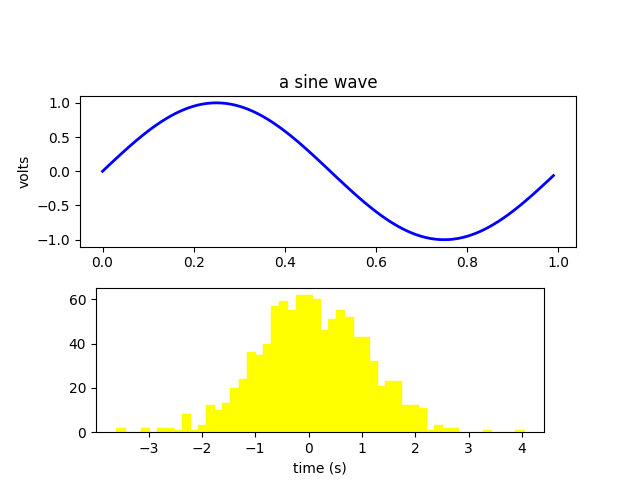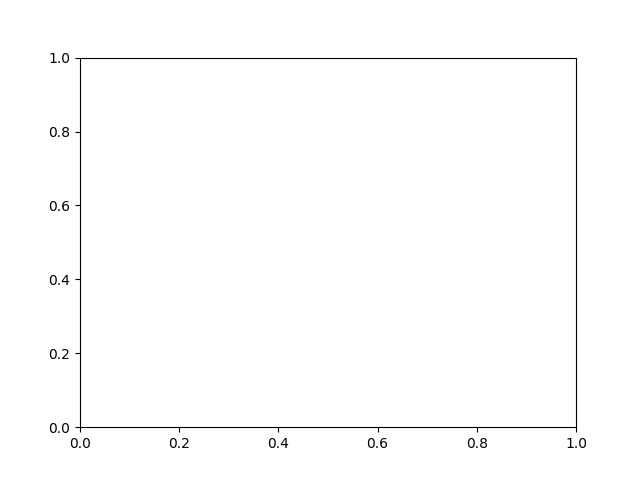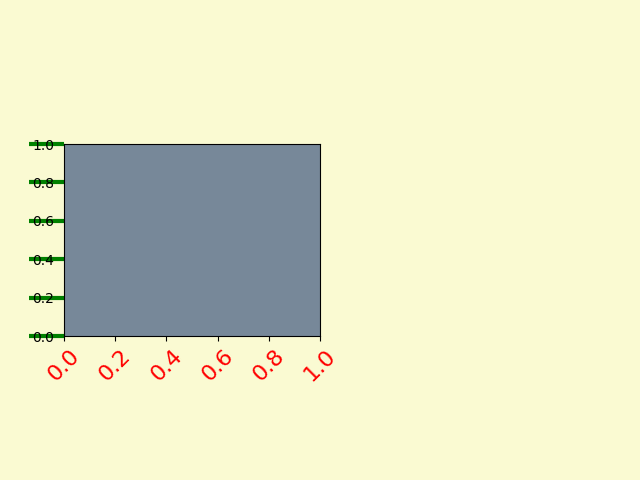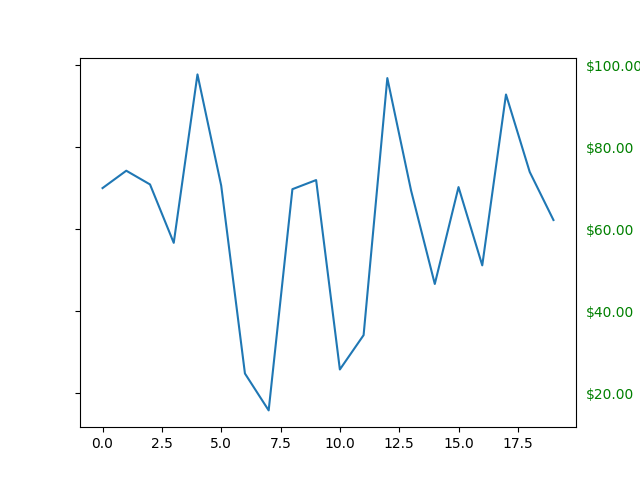# Artist tutorial¶

Using Artist objects to render on the canvas.

There are three layers to the matplotlib API.

• the matplotlib.backend_bases.FigureCanvas is the area onto which the figure is drawn
• the matplotlib.backend_bases.Renderer is the object which knows how to draw on the FigureCanvas
• and the matplotlib.artist.Artist is the object that knows how to use a renderer to paint onto the canvas.

The FigureCanvas and Renderer handle all the details of talking to user interface toolkits like wxPython or drawing languages like PostScript®, and the Artist handles all the high level constructs like representing and laying out the figure, text, and lines. The typical user will spend 95% of their time working with the Artists.

There are two types of Artists: primitives and containers. The primitives represent the standard graphical objects we want to paint onto our canvas: Line2D, Rectangle, Text, AxesImage, etc., and the containers are places to put them (Axis, Axes and Figure). The standard use is to create a Figure instance, use the Figure to create one or more Axes or Subplot instances, and use the Axes instance helper methods to create the primitives. In the example below, we create a Figure instance using matplotlib.pyplot.figure(), which is a convenience method for instantiating Figure instances and connecting them with your user interface or drawing toolkit FigureCanvas. As we will discuss below, this is not necessary -- you can work directly with PostScript, PDF Gtk+, or wxPython FigureCanvas instances, instantiate your Figures directly and connect them yourselves -- but since we are focusing here on the Artist API we'll let pyplot handle some of those details for us:

import matplotlib.pyplot as plt
fig = plt.figure()
ax = fig.add_subplot(2, 1, 1) # two rows, one column, first plot


The Axes is probably the most important class in the matplotlib API, and the one you will be working with most of the time. This is because the Axes is the plotting area into which most of the objects go, and the Axes has many special helper methods (plot(), text(), hist(), imshow()) to create the most common graphics primitives (Line2D, Text, Rectangle, AxesImage, respectively). These helper methods will take your data (e.g., numpy arrays and strings) and create primitive Artist instances as needed (e.g., Line2D), add them to the relevant containers, and draw them when requested. Most of you are probably familiar with the Subplot, which is just a special case of an Axes that lives on a regular rows by columns grid of Subplot instances. If you want to create an Axes at an arbitrary location, simply use the add_axes() method which takes a list of [left, bottom, width, height] values in 0-1 relative figure coordinates:

fig2 = plt.figure()
ax2 = fig2.add_axes([0.15, 0.1, 0.7, 0.3])


Continuing with our example:

import numpy as np
t = np.arange(0.0, 1.0, 0.01)
s = np.sin(2*np.pi*t)
line, = ax.plot(t, s, color='blue', lw=2)


In this example, ax is the Axes instance created by the fig.add_subplot call above (remember Subplot is just a subclass of Axes) and when you call ax.plot, it creates a Line2D instance and adds it to the Axes.lines list. In the interactive ipython session below, you can see that the Axes.lines list is length one and contains the same line that was returned by the line, = ax.plot... call:

In : ax.lines
Out: <matplotlib.lines.Line2D instance at 0x19a95710>

In : line
Out: <matplotlib.lines.Line2D instance at 0x19a95710>


If you make subsequent calls to ax.plot (and the hold state is "on" which is the default) then additional lines will be added to the list. You can remove lines later simply by calling the list methods; either of these will work:

del ax.lines
ax.lines.remove(line)  # one or the other, not both!


The Axes also has helper methods to configure and decorate the x-axis and y-axis tick, tick labels and axis labels:

xtext = ax.set_xlabel('my xdata') # returns a Text instance
ytext = ax.set_ylabel('my ydata')


When you call ax.set_xlabel, it passes the information on the Text instance of the XAxis. Each Axes instance contains an XAxis and a YAxis instance, which handle the layout and drawing of the ticks, tick labels and axis labels.

Try creating the figure below.

import numpy as np
import matplotlib.pyplot as plt

fig = plt.figure()
ax1.set_ylabel('volts')
ax1.set_title('a sine wave')

t = np.arange(0.0, 1.0, 0.01)
s = np.sin(2*np.pi*t)
line, = ax1.plot(t, s, color='blue', lw=2)

# Fixing random state for reproducibility
np.random.seed(19680801)

ax2 = fig.add_axes([0.15, 0.1, 0.7, 0.3])
n, bins, patches = ax2.hist(np.random.randn(1000), 50,
facecolor='yellow', edgecolor='yellow')
ax2.set_xlabel('time (s)')

plt.show()Every element in the figure is represented by a matplotlib Artist, and each has an extensive list of properties to configure its appearance. The figure itself contains a Rectangle exactly the size of the figure, which you can use to set the background color and transparency of the figures. Likewise, each Axes bounding box (the standard white box with black edges in the typical matplotlib plot, has a Rectangle instance that determines the color, transparency, and other properties of the Axes. These instances are stored as member variables Figure.patch and Axes.patch ("Patch" is a name inherited from MATLAB, and is a 2D "patch" of color on the figure, e.g., rectangles, circles and polygons). Every matplotlib Artist has the following properties

Property Description
alpha The transparency - a scalar from 0-1
animated A boolean that is used to facilitate animated drawing
axes The axes that the Artist lives in, possibly None
clip_box The bounding box that clips the Artist
clip_on Whether clipping is enabled
clip_path The path the artist is clipped to
contains A picking function to test whether the artist contains the pick point
figure The figure instance the artist lives in, possibly None
label A text label (e.g., for auto-labeling)
picker A python object that controls object picking
transform The transformation
visible A boolean whether the artist should be drawn
zorder A number which determines the drawing order
rasterized Boolean; Turns vectors into raster graphics (for compression & eps transparency)

Each of the properties is accessed with an old-fashioned setter or getter (yes we know this irritates Pythonistas and we plan to support direct access via properties or traits but it hasn't been done yet). For example, to multiply the current alpha by a half:

a = o.get_alpha()
o.set_alpha(0.5*a)


If you want to set a number of properties at once, you can also use the set method with keyword arguments. For example:

o.set(alpha=0.5, zorder=2)


If you are working interactively at the python shell, a handy way to inspect the Artist properties is to use the matplotlib.artist.getp() function (simply getp() in pyplot), which lists the properties and their values. This works for classes derived from Artist as well, e.g., Figure and Rectangle. Here are the Figure rectangle properties mentioned above:

In : matplotlib.artist.getp(fig.patch)
alpha = 1.0
animated = False
antialiased or aa = True
axes = None
clip_box = None
clip_on = False
clip_path = None
contains = None
edgecolor or ec = w
facecolor or fc = 0.75
figure = Figure(8.125x6.125)
fill = 1
hatch = None
height = 1
label =
linewidth or lw = 1.0
picker = None
transform = <Affine object at 0x134cca84>
verts = ((0, 0), (0, 1), (1, 1), (1, 0))
visible = True
width = 1
window_extent = <Bbox object at 0x134acbcc>
x = 0
y = 0
zorder = 1


The docstrings for all of the classes also contain the Artist properties, so you can consult the interactive "help" or the matplotlib.artist for a listing of properties for a given object.

## Object containers¶

Now that we know how to inspect and set the properties of a given object we want to configure, we need to know how to get at that object. As mentioned in the introduction, there are two kinds of objects: primitives and containers. The primitives are usually the things you want to configure (the font of a Text instance, the width of a Line2D) although the containers also have some properties as well -- for example the Axes Artist is a container that contains many of the primitives in your plot, but it also has properties like the xscale to control whether the xaxis is 'linear' or 'log'. In this section we'll review where the various container objects store the Artists that you want to get at.

### Figure container¶

The top level container Artist is the matplotlib.figure.Figure, and it contains everything in the figure. The background of the figure is a Rectangle which is stored in Figure.patch. As you add subplots (add_subplot()) and axes (add_axes()) to the figure these will be appended to the Figure.axes. These are also returned by the methods that create them:

In : fig = plt.figure()

In : ax2 = fig.add_axes([0.1, 0.1, 0.7, 0.3])

In : ax1
Out: <matplotlib.axes.Subplot instance at 0xd54b26c>

In : print(fig.axes)
[<matplotlib.axes.Subplot instance at 0xd54b26c>, <matplotlib.axes.Axes instance at 0xd3f0b2c>]


Because the figure maintains the concept of the "current axes" (see Figure.gca and Figure.sca) to support the pylab/pyplot state machine, you should not insert or remove axes directly from the axes list, but rather use the add_subplot() and add_axes() methods to insert, and the delaxes() method to delete. You are free however, to iterate over the list of axes or index into it to get access to Axes instances you want to customize. Here is an example which turns all the axes grids on:

for ax in fig.axes:
ax.grid(True)


The figure also has its own text, lines, patches and images, which you can use to add primitives directly. The default coordinate system for the Figure will simply be in pixels (which is not usually what you want) but you can control this by setting the transform property of the Artist you are adding to the figure.

More useful is "figure coordinates" where (0, 0) is the bottom-left of the figure and (1, 1) is the top-right of the figure which you can obtain by setting the Artist transform to fig.transFigure:

import matplotlib.lines as lines

fig = plt.figure()

l1 = lines.Line2D([0, 1], [0, 1], transform=fig.transFigure, figure=fig)
l2 = lines.Line2D([0, 1], [1, 0], transform=fig.transFigure, figure=fig)
fig.lines.extend([l1, l2])

plt.show()Here is a summary of the Artists the figure contains

Figure attribute Description
axes A list of Axes instances (includes Subplot)
patch The Rectangle background
images A list of FigureImages patches - useful for raw pixel display
legends A list of Figure Legend instances (different from Axes.legends)
lines A list of Figure Line2D instances (rarely used, see Axes.lines)
patches A list of Figure patches (rarely used, see Axes.patches)
texts A list Figure Text instances

### Axes container¶

The matplotlib.axes.Axes is the center of the matplotlib universe -- it contains the vast majority of all the Artists used in a figure with many helper methods to create and add these Artists to itself, as well as helper methods to access and customize the Artists it contains. Like the Figure, it contains a Patch patch which is a Rectangle for Cartesian coordinates and a Circle for polar coordinates; this patch determines the shape, background and border of the plotting region:

ax = fig.add_subplot(111)
rect = ax.patch  # a Rectangle instance
rect.set_facecolor('green')


When you call a plotting method, e.g., the canonical plot() and pass in arrays or lists of values, the method will create a matplotlib.lines.Line2D() instance, update the line with all the Line2D properties passed as keyword arguments, add the line to the Axes.lines container, and returns it to you:

In : x, y = np.random.rand(2, 100)

In : line, = ax.plot(x, y, '-', color='blue', linewidth=2)


plot returns a list of lines because you can pass in multiple x, y pairs to plot, and we are unpacking the first element of the length one list into the line variable. The line has been added to the Axes.lines list:

In : print(ax.lines)
[<matplotlib.lines.Line2D instance at 0xd378b0c>]


Similarly, methods that create patches, like bar() creates a list of rectangles, will add the patches to the Axes.patches list:

In : n, bins, rectangles = ax.hist(np.random.randn(1000), 50, facecolor='yellow')

In : rectangles
Out: <a list of 50 Patch objects>

In : print(len(ax.patches))


You should not add objects directly to the Axes.lines or Axes.patches lists unless you know exactly what you are doing, because the Axes needs to do a few things when it creates and adds an object. It sets the figure and axes property of the Artist, as well as the default Axes transformation (unless a transformation is set). It also inspects the data contained in the Artist to update the data structures controlling auto-scaling, so that the view limits can be adjusted to contain the plotted data. You can, nonetheless, create objects yourself and add them directly to the Axes using helper methods like add_line() and add_patch(). Here is an annotated interactive session illustrating what is going on:

In : fig, ax = plt.subplots()

# create a rectangle instance
In : rect = matplotlib.patches.Rectangle((1, 1), width=5, height=12)

# by default the axes instance is None
In : print(rect.axes)
None

# and the transformation instance is set to the "identity transform"
In : print(rect.get_transform())
<Affine object at 0x13695544>

# now we add the Rectangle to the Axes

# and notice that the ax.add_patch method has set the axes
# instance
In : print(rect.axes)
Axes(0.125,0.1;0.775x0.8)

# and the transformation has been set too
In : print(rect.get_transform())
<Affine object at 0x15009ca4>

# the default axes transformation is ax.transData
In : print(ax.transData)
<Affine object at 0x15009ca4>

# notice that the xlimits of the Axes have not been changed
In : print(ax.get_xlim())
(0.0, 1.0)

# but the data limits have been updated to encompass the rectangle
In : print(ax.dataLim.bounds)
(1.0, 1.0, 5.0, 12.0)

# we can manually invoke the auto-scaling machinery
In : ax.autoscale_view()

# and now the xlim are updated to encompass the rectangle
In : print(ax.get_xlim())
(1.0, 6.0)

# we have to manually force a figure draw
In : ax.figure.canvas.draw()


There are many, many Axes helper methods for creating primitive Artists and adding them to their respective containers. The table below summarizes a small sampling of them, the kinds of Artist they create, and where they store them

Helper method Artist Container
ax.annotate - text annotations Annotate ax.texts
ax.bar - bar charts Rectangle ax.patches
ax.errorbar - error bar plots Line2D and Rectangle ax.lines and ax.patches
ax.fill - shared area Polygon ax.patches
ax.hist - histograms Rectangle ax.patches
ax.imshow - image data AxesImage ax.images
ax.legend - axes legends Legend ax.legends
ax.plot - xy plots Line2D ax.lines
ax.scatter - scatter charts PolygonCollection ax.collections
ax.text - text Text ax.texts

In addition to all of these Artists, the Axes contains two important Artist containers: the XAxis and YAxis, which handle the drawing of the ticks and labels. These are stored as instance variables xaxis and yaxis. The XAxis and YAxis containers will be detailed below, but note that the Axes contains many helper methods which forward calls on to the Axis instances so you often do not need to work with them directly unless you want to. For example, you can set the font color of the XAxis ticklabels using the Axes helper method:

for label in ax.get_xticklabels():
label.set_color('orange')


Below is a summary of the Artists that the Axes contains

Axes attribute Description
artists A list of Artist instances
patch Rectangle instance for Axes background
collections A list of Collection instances
images A list of AxesImage
legends A list of Legend instances
lines A list of Line2D instances
patches A list of Patch instances
texts A list of Text instances
xaxis matplotlib.axis.XAxis instance
yaxis matplotlib.axis.YAxis instance

### Axis containers¶

The matplotlib.axis.Axis instances handle the drawing of the tick lines, the grid lines, the tick labels and the axis label. You can configure the left and right ticks separately for the y-axis, and the upper and lower ticks separately for the x-axis. The Axis also stores the data and view intervals used in auto-scaling, panning and zooming, as well as the Locator and Formatter instances which control where the ticks are placed and how they are represented as strings.

Each Axis object contains a label attribute (this is what pyplot modifies in calls to xlabel() and ylabel()) as well as a list of major and minor ticks. The ticks are XTick and YTick instances, which contain the actual line and text primitives that render the ticks and ticklabels. Because the ticks are dynamically created as needed (e.g., when panning and zooming), you should access the lists of major and minor ticks through their accessor methods get_major_ticks() and get_minor_ticks(). Although the ticks contain all the primitives and will be covered below, Axis instances have accessor methods that return the tick lines, tick labels, tick locations etc.:

fig, ax = plt.subplots()
axis = ax.xaxis
axis.get_ticklocs()Out:

array([0. , 0.2, 0.4, 0.6, 0.8, 1. ])

axis.get_ticklabels()


Out:

<a list of 6 Text major ticklabel objects>


note there are twice as many ticklines as labels because by default there are tick lines at the top and bottom but only tick labels below the xaxis; however, this can be customized.

axis.get_ticklines()


Out:

<a list of 12 Line2D ticklines objects>


And with the above methods, you only get lists of major ticks back by default, but you can also ask for the minor ticks:

axis.get_ticklabels(minor=True)
axis.get_ticklines(minor=True)


Out:

<a list of 0 Line2D ticklines objects>


Here is a summary of some of the useful accessor methods of the Axis (these have corresponding setters where useful, such as set_major_formatter().)

Accessor method Description
get_scale The scale of the axis, e.g., 'log' or 'linear'
get_view_interval The interval instance of the axis view limits
get_data_interval The interval instance of the axis data limits
get_gridlines A list of grid lines for the Axis
get_label The axis label - a Text instance
get_ticklabels A list of Text instances - keyword minor=True|False
get_ticklines A list of Line2D instances - keyword minor=True|False
get_ticklocs A list of Tick locations - keyword minor=True|False
get_major_locator The matplotlib.ticker.Locator instance for major ticks
get_major_formatter The matplotlib.ticker.Formatter instance for major ticks
get_minor_locator The matplotlib.ticker.Locator instance for minor ticks
get_minor_formatter The matplotlib.ticker.Formatter instance for minor ticks
get_major_ticks A list of Tick instances for major ticks
get_minor_ticks A list of Tick instances for minor ticks
grid Turn the grid on or off for the major or minor ticks

Here is an example, not recommended for its beauty, which customizes the axes and tick properties

# plt.figure creates a matplotlib.figure.Figure instance
fig = plt.figure()
rect = fig.patch  # a rectangle instance
rect.set_facecolor('lightgoldenrodyellow')

ax1 = fig.add_axes([0.1, 0.3, 0.4, 0.4])
rect = ax1.patch
rect.set_facecolor('lightslategray')

for label in ax1.xaxis.get_ticklabels():
# label is a Text instance
label.set_color('red')
label.set_rotation(45)
label.set_fontsize(16)

for line in ax1.yaxis.get_ticklines():
# line is a Line2D instance
line.set_color('green')
line.set_markersize(25)
line.set_markeredgewidth(3)

plt.show()### Tick containers¶

The matplotlib.axis.Tick is the final container object in our descent from the Figure to the Axes to the Axis to the Tick. The Tick contains the tick and grid line instances, as well as the label instances for the upper and lower ticks. Each of these is accessible directly as an attribute of the Tick.

Tick attribute Description
tick1line Line2D instance
tick2line Line2D instance
gridline Line2D instance
label1 Text instance
label2 Text instance

Here is an example which sets the formatter for the right side ticks with dollar signs and colors them green on the right side of the yaxis

import matplotlib.ticker as ticker

# Fixing random state for reproducibility
np.random.seed(19680801)

fig, ax = plt.subplots()
ax.plot(100*np.random.rand(20))

formatter = ticker.FormatStrFormatter('\$%1.2f')
ax.yaxis.set_major_formatter(formatter)

for tick in ax.yaxis.get_major_ticks():
tick.label1.set_visible(False)
tick.label2.set_visible(True)
tick.label2.set_color('green')

plt.show()Keywords: matplotlib code example, codex, python plot, pyplot Gallery generated by Sphinx-Gallery